Function Repository Resource:

# SalzerPiessensInversionWeights

Get a list of abscissas and weights for the numerical inverse Laplace transform

Contributed by: Jan Mangaldan
 ResourceFunction["SalzerPiessensInversionWeights"][n] gives a list of the 2 n+1 triples {xi,wi,εi} of the (2 n+1)-point Salzer-Piessens formula for numerical Laplace transform inversion, where wi and εi are the corresponding weight and error weight of the abscissa xi. ResourceFunction["SalzerPiessensInversionWeights"][n,prec] uses the working precision prec.

## Details

Salzer-Piessens approximates the inverse Laplace transform as a linear combination of values of the function to be transformed, evaluated at abscissas xi: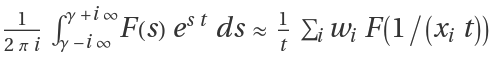.
An error estimate of the result can be computed using a similar formula, but using the error weights εi in place of the weights wi: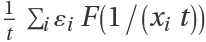.
The Salzer-Piessens method is based on the roots of the nth order Bessel polynomial comprising n of the abscissas for a Gaussian quadrature rule. An n+1 Kronrod-type extension is then built out of this Gaussian rule.
The error estimate is equivalent to the difference between the complete (2 n+1)-point approximation and the n-point approximation from the original Gaussian rule.
The precision argument acts similarly to the WorkingPrecision option used in many Wolfram Language numeric functions; it is not at all like the PrecisionGoal option. If n is too small to allow for a high-precision result, extra precision in a result will not be meaningful.

## Examples

### Basic Examples (2)

The abscissas, weights and error weights for a 7-point Salzer-Piessens rule:

 In:=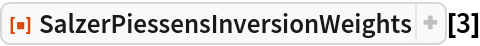Out=Use the specified precision:

 In:=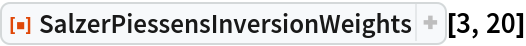Out=### Applications (7)

A function to be transformed:

 In:=Generate a 21-point Salzer-Piessens rule at high precision:

 In:=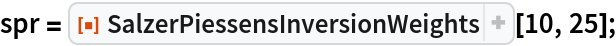Numerically evaluate its inverse Laplace transform at a given point:

 In:=Out=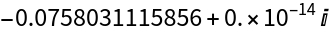Compute the error estimate:

 In:=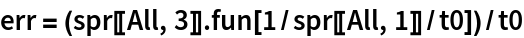Out=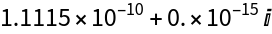In:=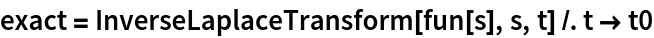Out=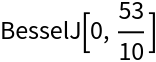In:=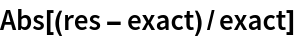Out=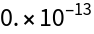Plot the exact and approximate transform together:

 In:=Out=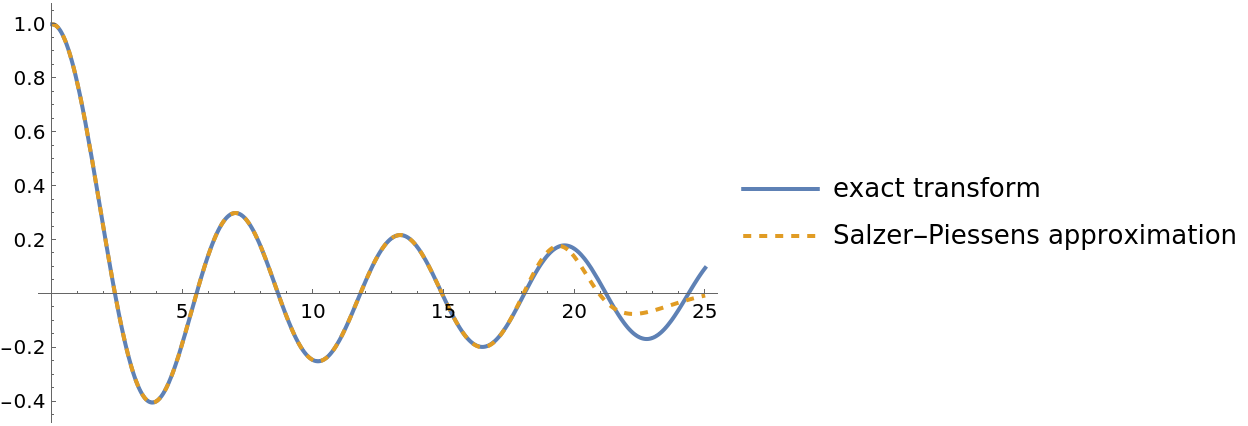Plot the error estimate:

 In:=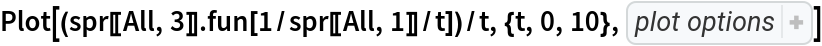Out=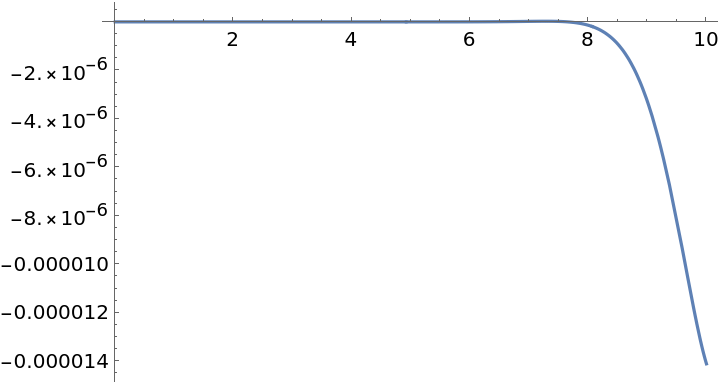### Properties and Relations (1)

A (2 n+1)-point Salzer-Piessens rule gives the exact inverse Laplace transform for functions of the form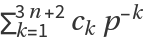:

 In:=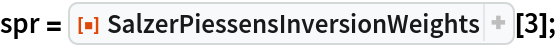In:=Out=In:=Out=### Possible Issues (3)

A function to be transformed:

 In:=Machine precision usually does not give sufficient accuracy due to the ill-conditioned nature of numerical Laplace transform inversion:

 In:=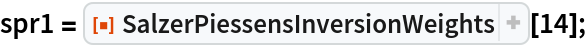In:=Out=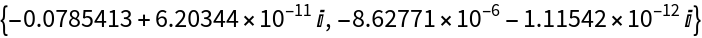Arbitrary precision is often necessary to get accurate results:

 In:=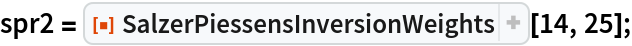In:=Out=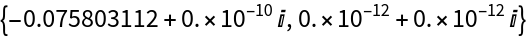## Version History

• 1.0.0 – 23 March 2021